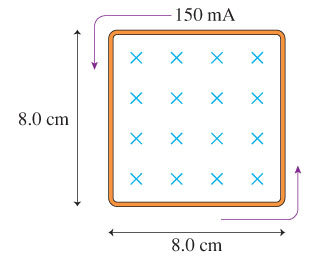# Problem: The loop in the figure has an induced current as shown. The loop has a resistance of 0.24 Ω.Part A: Is the magnetic field strength increasing or decreasing?Part B: What is the rate of change of the field, ΔB/Δt?|ΔB/Δt| = _______ T/sExpress your answer using two significant figures.

###### FREE Expert Solution

Part A

The induced current is in the counter-clockwise direction.

The current is in the opposite direction to the change in flux.

Thus, the flux is increasing into the page direction.

90% (20 ratings)###### Problem Details

The loop in the figure has an induced current as shown. The loop has a resistance of 0.24 Ω.Part A: Is the magnetic field strength increasing or decreasing?

Part B: What is the rate of change of the field, ΔB/Δt?

|ΔB/Δt| = _______ T/s